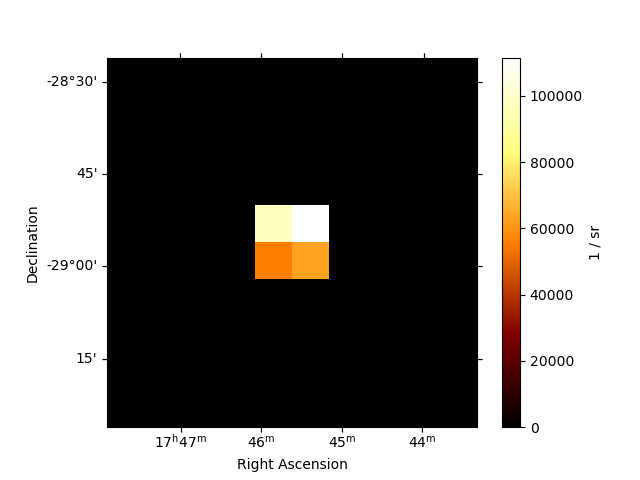# Point spatial model¶

This model is a delta function centered in lon_0 and lat_0 parameters provided:

$\phi(lon, lat) = \delta{(lon - lon_0, lat - lat_0)}$

The model is defined on the celestial sphere in the coordinate frame provided by the user.

If the point source is not centered on a pixel, the flux is re-distributed across 4 neighbouring pixels. This ensured that the center of mass position is conserved.

## Example plot¶

Here is an example plot of the model:

from astropy.coordinates import SkyCoord
from gammapy.maps import WcsGeom
from gammapy.modeling.models import (
Models,
PointSpatialModel,
PowerLawSpectralModel,
SkyModel,
)

model = PointSpatialModel(
lon_0="0.01 deg",
lat_0="0.01 deg",
frame="galactic",
)

geom = WcsGeom.create(
skydir=SkyCoord("0d 0d", frame="galactic"), width=(1, 1), binsz=0.1
)## YAML representation¶

Here is an example YAML file using the model:

pwl = PowerLawSpectralModel()
point = PointSpatialModel()

model = SkyModel(spectral_model=pwl, spatial_model=point, name="pwl-point-model")
models = Models([model])

print(models.to_yaml())


Out:

components:
-   name: pwl-point-model
type: SkyModel
spectral:
type: PowerLawSpectralModel
parameters:
-   name: index
value: 2.0
-   name: amplitude
value: 1.0e-12
unit: cm-2 s-1 TeV-1
-   name: reference
value: 1.0
unit: TeV
frozen: true
spatial:
type: PointSpatialModel
frame: icrs
parameters:
-   name: lon_0
value: 0.0
unit: deg
-   name: lat_0
value: 0.0
unit: deg


Gallery generated by Sphinx-Gallery